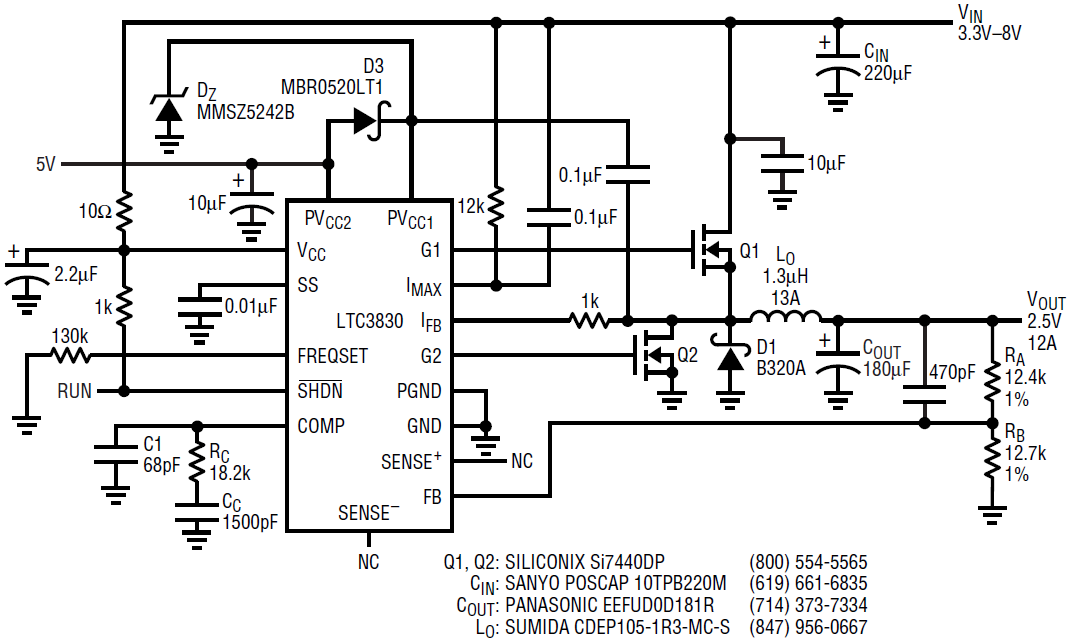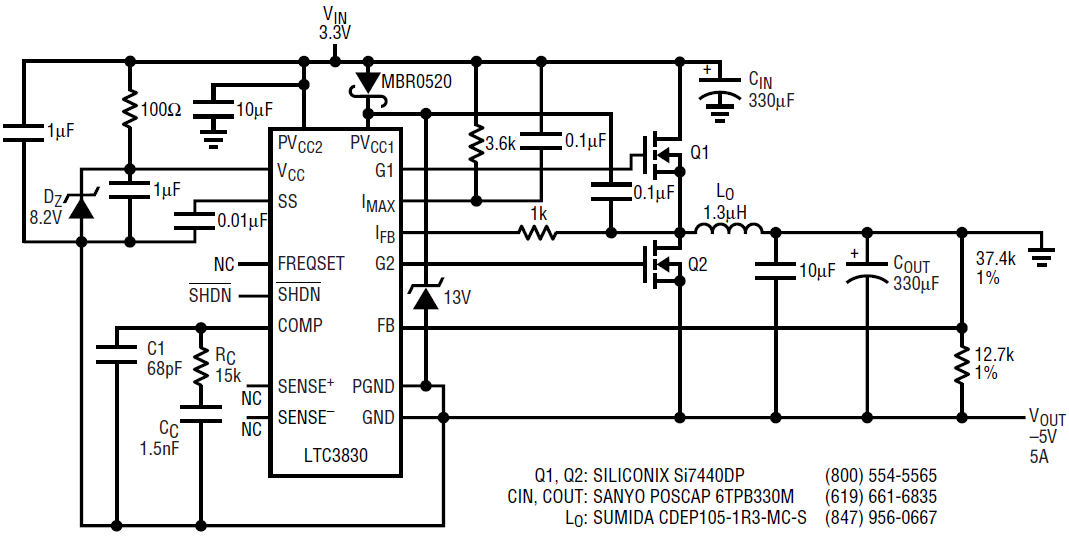# Versatile LTC3830 and LTC3832 Deliver High Efficiency for Step-Down, Step-Up and Inverting Power Conversions

Introduction

The LTC3830 and the LTC3832 are pin-to-pin compatible upgrades to the LTC1430—a popular IC for low voltage step-down applications due to its simplicity and high efficiency. The LTC3830 and the LTC3832 remove the LTC1430’s frequency foldback at startup, thus eliminating inrush current and resulting output overshoot. Other improvements over the LTC1430 include tighter gm distribution of the error amplifier and tighter current limiting. The LTC3832 is identical to the LTC3830, except that it incorporates a 0.6V reference for the output feedback, a larger gm and a default frequency of 300kHz (instead of the 200kHz for the LTC3830), making it good match for very low output applications. The higher frequency of the LTC3832 also allows the use of smaller inductors and capacitors, making for a smaller overall solution.

This article shows several designs using the LTC3830 for step down, step up and inverting applications. The LTC3832 can be used in place of the LTC3830 in any of these designs. All that is required are some minor adjustments to the feedback resistor divider and the compensation RC component values.

12A High Efficiency Step Down Power Supply Converts 3.3V–8V Input to a 2.5V Output

LTC3830/3832 are voltage mode synchronous buck controllers with two powerful MOSFET drivers for both the main MOSFET and a synchronous MOSFET. The RDS(ON) of the main MOSFET is used to establish the current limit, thus eliminating the sense resistor and its associated power loss. The current limit and switching frequency can be programmed easily through external resistors.

Figure 1 shows the schematic diagram of a 12A step down design based on LTC3830. The input is 3.3V to 8V and the output is 2.5V. To obtain different output voltages, vary the ratio of RA/RB. With only two tiny PowerPak SO8 MOSFETs and 300kHz switching frequency, this design achieves close to 90% efficiency with 5V input and 2.5V output. The overall footprint of this design is less than 1"×1.2", with all of the components placed on the same side of the board. For higher output currents, simply parallel more MOSFETs and use an inductor with a higher current rating.Figure 1. Schematic diagram of 2.5V/12A synchronous step-down power supply

5A Step Up Power Supply Converts 3.3V to 5V

Although intended for synchronous buck applications, LTC3830 and LTC3832 can also be used in other circuit topologies. Figure 2a shows a synchronous boost design using LTC3830 converting 3.3V to 5V. Compared to a conventional boost converter, this design uses a low RDS(ON) N-channel MOSFET to implement the synchronous rectification, therefore improving efficiency by 5% to 10%. The maximum output current is 8A with only two PowerPak SO8 MOSFETs. A current sense resistor is used for more accurate current limiting than can be achieved by sensing RDS(ON) of the MOSFET. One may also use the DCR of the inductor to implement the current limit function, as shown in Figure 2b. RF and CF filters out the AC voltage components of the inductor voltage to obtain the DC voltage drop on the DC resistance of the inductor. This scheme eliminates the sense resistor and its associated power loss, but the response to overcurrent conditions is slower than a topology that uses a sense resistor. The delay time is determined by the product of RF • CF.Figure 2a. Schematic diagram of 3.3V to 5V synchronous boost converterFigure 2b. How to use the DC resistance of the boost inductor to control current limiting

5A Inverter Converts 3.3V to –5V

The LTC3830 and LTC3832 can also be used in inverting applications. Figure 3 shows a synchronous buck-boost power supply which converts 3.3V into –5V. The total VCC supply voltage in this design is the sum of the absolute values of input and output voltages, which is about 8.3V; and the PVCC1 voltage is the VCC voltage plus 5V, which is 13.3V. Since these voltage stresses are very close to the maximum voltage ratings for the LTC3830 and the LTC3832 (VCC(MAX) = 9V and PVCC1(MAX) = 14V), Zener diodes should be placed on VCC and PVCC1 pins to provide overvoltage protection.Figure 3. Schematic diagram of 3.3V to –5V inverting converter

Conclusion

The LTC3830 and LTC3832 are versatile voltage mode controllers that can be used in variety of applications including step up, step down and voltage inversion. Their integrated high current MOSFET drivers and programmable frequencies allow users to minimize power loss and total solution size.